# Python While Loops Tutorial

Learn how while loop works in Python.

The while loop is somewhat similar to an `if` statement, it executes the code inside, if the condition is True. However, as opposed to the `if` statement, the while loop continues to execute the code repeatedly as long as the condition is True.

## The while Loop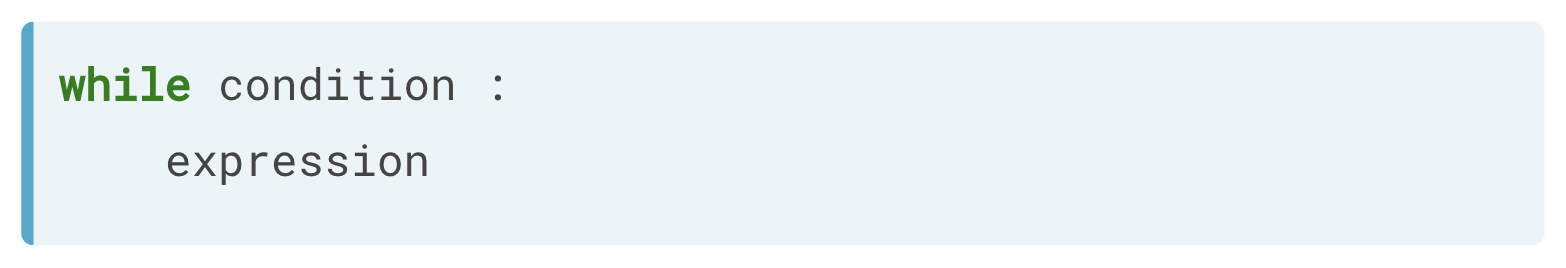The syntax of the while loop is very similar to the if statement, as you can see above. The while loop is not very common, but in some cases, it can be very useful.

### Example

Suppose you are numerically calculating a model based on your data. This will typically involve taking the same steps repeatedly until the error between your model and your data is below some boundary. When you can reformulate the problem as `repeating an action until a condition is met`, a while loop is often the way to go.

In the below example, you initially start with an error equal to `50.0`. Next, you will write a while loop, in the condition part, we write error > 1, so that the while loop executes again as long as the error is above 1. Inside the while loop, you divide the error by four and update the error variable. When we run it the first time, we receive 12.5.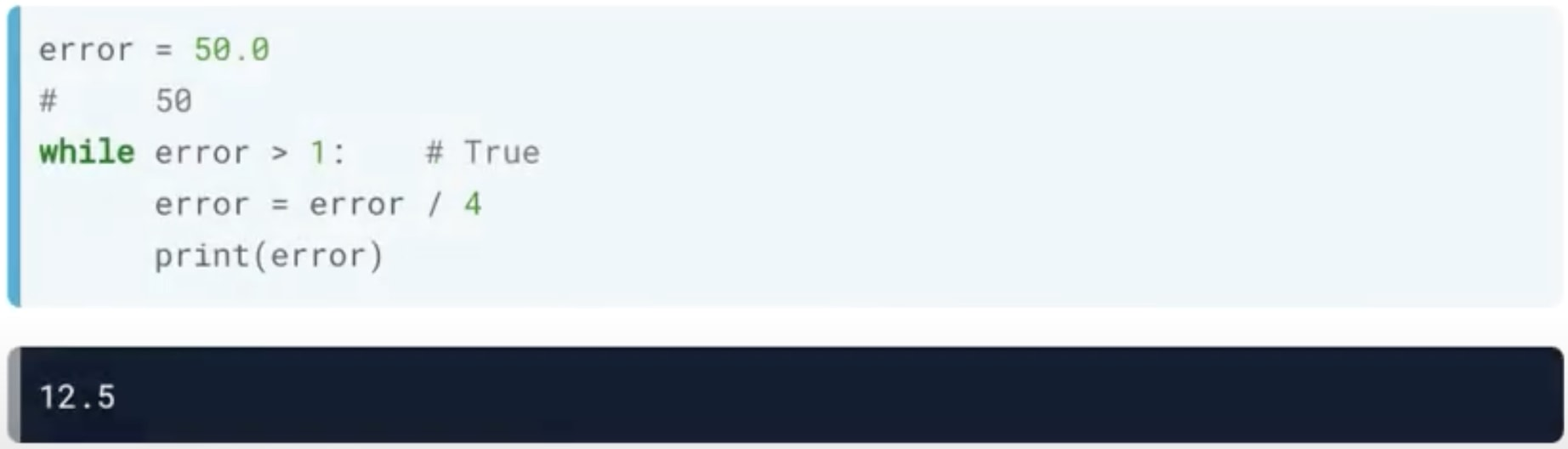Python will then go back to the condition of the while loop with the new error equal to 12.5. The condition will again be true, and the code is executed.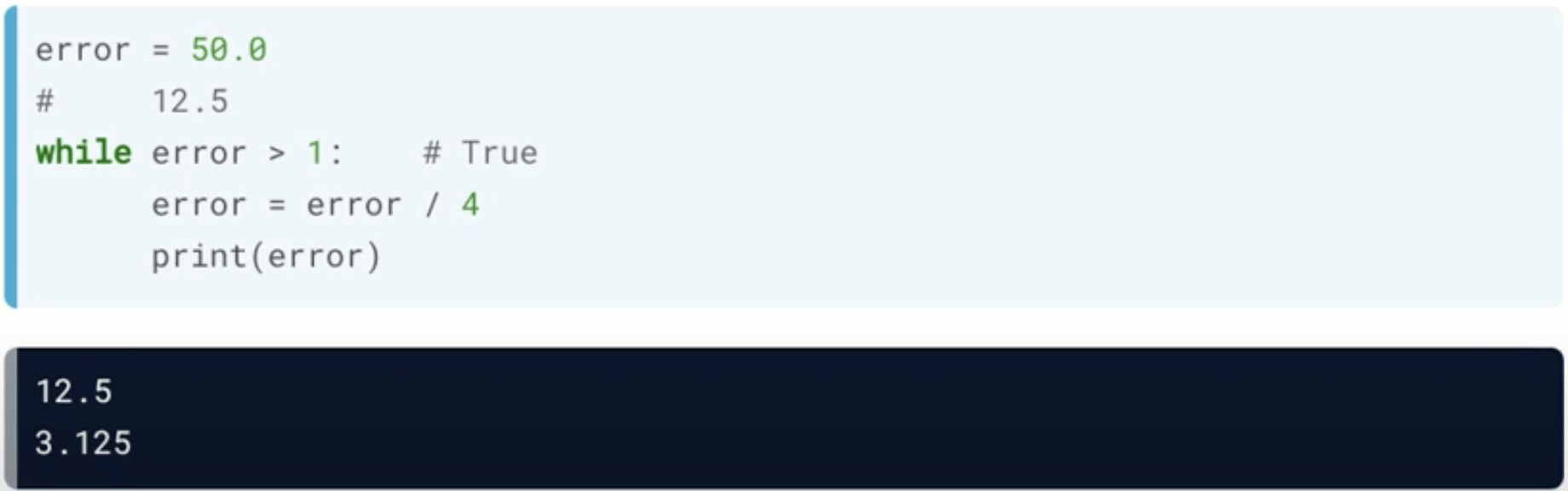Python will again move back to the condition of the while loop and attempt to re-run the code. Because 3.125 is greater than 1, the code makes the calculation.Finally, the new condition of the while loop is less than 1, so the code results in an error and won't run.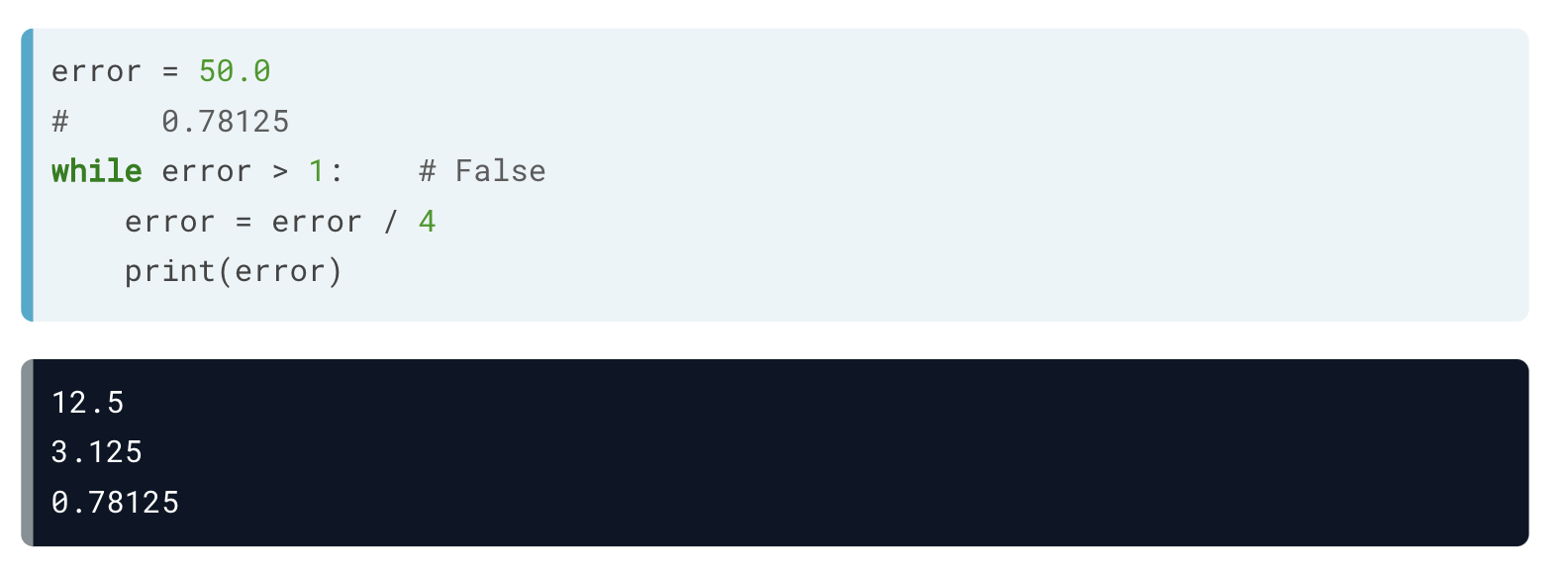This example will come in handy because it's time to build a `while` loop yourself! We're going to code a `while` loop that implements a very basic control system for an inverted pendulum. If there's an offset from standing perfectly straight, the `while` loop will incrementally fix this offset.

Note that if your `while` loop takes too long to run, you might have made a mistake. In particular, remember to indent the contents of the loop!

### Example of While Loop Error (Continuously Running)

Suppose you were to comment out or remove the error value's updates and rerun the script. The error won't be updated, and the condition will always be true. This will result in the while loop running forever.If you were to make this mistake on your system, you would have to interrupt the Python program by pressing the Control + C keys.

### Example

In the below example, you will create the variable `offset` with an initial value of `8`. Then you will write a `while` loop that keeps running as long as the `offset` is not equal to `0`.

Inside the `while` loop:

• You will print out the sentence `"correcting..."`.
• Next, decrease the value of `offset` by 1. You can do this with `offset = offset - 1`.
• Finally, within your while loop, print out `offset` so you can see how it changes.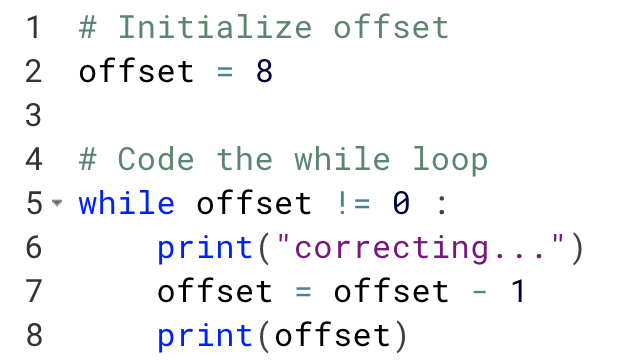When we run the above program, it produces the following result:

``````correcting...
7
correcting...
6
correcting...
5
correcting...
4
correcting...
3
correcting...
2
correcting...
1
correcting...
0
``````